International Journal of Fluid Mechanics & Thermal Sciences
Volume 1, Issue 1, April 2015, Pages: 1-7

Fluid Dynamic Investigation of the Height Effect of an Inclined Roof Obstacle

Slah Driss, Zied Driss, Imen Kallel Kammoun

Laboratory of Electro-Mechanic Systems (LASEM), National School of Engineers of Sfax (ENIS), Univrsity of Sfax, Sfax, Tunisia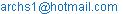(S. Driss)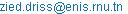(Z. Driss)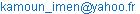(I. K. Kammoun)

Slah Driss, Zied Driss, Imen Kallel Kammoun. Fluid Dynamic Investigation of the Height Effect of an Inclined Roof Obstacle. International Journal of Fluid Mechanics & Thermal Sciences. Vol. 1, No. 1, 2015, pp. 1-7. doi: 10.11648/j.ijfmts.20150101.11

Abstract: The present paper is dedicated to the numerical simulation of the height effect of an inclined roof obstacle. The governing equations of mass and momentum in conjunction with the standard k-ε turbulence model are solved using the computational fluid Dynamics (CFD). The numerical method used a finite volume discretization. Experiments in wind tunnel are also developed to measure the average velocity near two inclined roof obstacles. The numerical simulations agreed reasonably with the experimental results and the numerical model was validated.

Keywords: Fluid Dynamic, Height Effect, Inclined Roof Obstacle, Wind Tunnel

1. Introduction

Air quality within an urban street canyon is influenced by traffic flow and its emissions, urban background concentrations, ambient meteorological parameters, and building geometry configurations such as roof shape, building height, street width, etc. In this context, Nozawa  studied the turbulent inflow data generated for both a smooth surface and rough surfaces lyres. Ikhwan et al.  conducted in a boundary layer wind tunnel. The velocities of the flow around the pyramids and the pressure distribution on the pyramid surfaces were measured using 2D laser Doppler anemometry (LDA) and standard pressure tapping technique, respectively. Eight different pyramids with varying base angles were investigated. The mean and fluctuating characteristics that distinguish pyramids field around pyramids surfaces are described. Ahmad et al.  provided a comprehensive literature on wind tunnel simulation studies in urban street including the effects of building configurations, canyon geometries, traffic induced turbulence and variable approaching wind directions on flow fields and exhaust dispersion. Melo et al.  developed two Gaussian atmospheric dispersion models, AERMOD and CALPUFF. Both incorporating the PRIME algorithm for plume rise and building downwash, are intercompared and validated using wind tunnel data on odour dispersion around a complex pig farm facility comprising of two attached buildings. The results show that concentrations predicted by AERMOD are in general higher than those predicted by CALPUFF, especially regarding the maximum mean concentrations observed in the near field. Comparison of the model results with wind tunnel data showed that both models adequately predict mean concentrations further downwind from the facility. However, closer to the buildings, the models may over-predict or under-predict concentrations by a factor of two, and in certain cases even larger, depending on the conditions. Tominaga and Stathopoulos  reviewed current modeling techniques in CFD simulation of near-field pollutant dispersion in urban environments and discussed the findings to give insight into future applications. Ould Said et al.  dedicated to the numerical simulation of thermal convection in a two dimensional vertical conical cylinder partially annular space. The governing equations of mass, momentum and energy are solved using the CFD code "FLUENT". The results of streamlines and the isotherms of the fluid are discussed for different annuli with various boundary conditions and Rayleigh numbers. Princevac et al.  observed and quantitatively measured, within regular 3x3 and 5x5 arrays of cubes, a sideways mean inflow in the lee of all succeeding rows of buildings. When the central building in a 3x3 array is replaced by a building of double height, due to the strong downdraft caused by this tall building, the lateral outflow becomes significantly more intense. Lateb et al.  determined the turbulence model that best helped reproduce pollutant plume dispersion. The most critical case was considered, namely, when wind blew perpendicularly towards the upstream tower, then placing the building in its wake. When numerical results were compared to wind tunnel experiments, it was found that the realizable k-ε turbulence model yielded the best agreement with wind tunnel results for the lowest stack height. For the highest stack height, the RNG k-ε turbulence model provided greater concordance with experimental results. Vizotto  developed a computational model of free-form shell generation in the design of roof structures that rely on the optimized behaviour of the membrane theory of thin shells. For architects and engineers, the model offers a low cost, fast, and relatively easy design solution. Jiang et al.  studied three ventilation cases, single-sided ventilation with an opening in windward wall, single-sided ventilation with an opening in leeward wall, and cross ventilation. In the wind tunnel, a laser Doppler anemometry was used to provide accurate and detailed velocity data. In LES calculations, two subgrid-scale (SS) models, a Smagorinsky SS model and a filtered dynamic SS model, were used. The numerical results from LES are in good agreement with the experimental data, in particular with the predicted airflow patterns. Fabien et al.  developed a theoretical model for the calculation of driving rain by which the infuence of wind, building geometry and rainfall on the driving-rain intensity distributions on building envelopes can be studied. In this model a catch ratio is introduced which depends on raindrop diameter, reference wind speed in the undisturbed wind ﬂow, on building geometry and on the position on the building envelope. By the use of computational ﬂuid dynamics catch ratios for several wind speeds and geometries have been calculated. Driving-rain intensities and its distribution on the envelope can be calculated with a chosen raindrop spectrum. Lim et al.  presented a numerical simulation of flow around a surface mounted cube placed in a turbulent boundary layer which, although representing a typical wind environment, to match a series of wind tunnel observations. The simulations were carried out at a Reynolds number, based on the velocity at the cube height, of 20,000. The results presented include detailed comparison between measurements and LES computations of both the inflow boundary layer and the flow field around the cube including mean and fluctuating surface pressures. Ntinas et al.  predicted the airflow around buildings. A time-dependent simulation model has been applied for the prediction of the turbulent airflow around obstacles with arched and pitched roof geometry, under wind tunnel conditions. To verify the reliability of the model, an experiment was conducted inside a wind tunnel and the air velocity and turbulent kinetic energy profiles were measured around two small-scale obstacles with an arched-type and a pitched-type roof. Luo et al.  studied models of cuboid obstacles to characterize the three-dimensional responses of airflow behind obstacles with different shape ratios to variations in the incident flow in a wind-tunnel simulation. Wind velocity was measured using particle image velocimetry (PIV). The flow patterns behind cuboid obstacles were complicated by changes in the incidence angle of the approaching flow and in the obstacle's shape ratio.

According to these anterior studies, it’s clear that the study of the aerodynamic around the obstacle is very interesting. Indeed, the literature review confirms that there is paucity on the inclined roof obstacle study. For thus, we are interested on the study of the height effect of an inclined roof obstacle.

2. Numerical Model

2.1. Geometrical Parameters

The geometrical parameters of the obstacle with inclined roof shape are presented in figure 1. This model is characterized by a length equal to l=118 mm and a first height equal to b=82 mm. The second height is equal to h=H=153 mm in the first case and to h=2H=306 mm in the second case. The computational domain is shown in figure 2. It is defined by the interior volume of the wind tunnel blocked by two planes. The first one is in the tranquillization chamber entry and the second one is in the exit of the diffuser.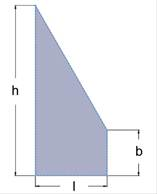Figure 1. Geometrical arrangement.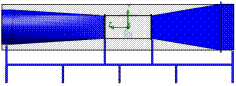(a) h=H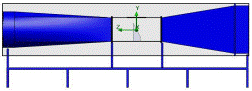(b) h=2 H

Figure 2. Computational domain.

2.2. Boundary Conditions

A boundary condition is required any where fluid enters the system and can be set as a pressure, mass flow, volume flow or velocity. For the inlet velocity, we will have imposed a value of 0.9 m.s-1 and for the outlet pressure a value of 101325 Pa which means that at this opening the fluid exits the model to an area of atmospheric pressure. Aware that the obstacle is suspended in our domain, both the roof top obstacle and the wall of our domain are considered as a wall boundary condition (figure 3).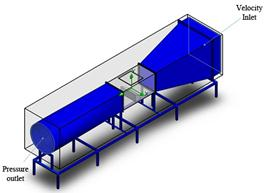High value (mm) Velocity inlet (m.s-1) Pressure outlet (Pa) 153 0.9 101325 306

Figure 3. Boundary conditions.

2.3. Mesh Resolution

Figure 4 shows the initial mesh of the model. It is named initial since it is the mesh that the calculation starts from and it could be further refined during the calculation if the solution-adaptive meshing is enabled. The initial mesh is constructed from the basic mesh by refining the basic mesh cells in accordance with the specified mesh settings. The basic mesh is formed by dividing the computational domain into slices by parallel planes which are orthogonal to the global coordinate system’s axes.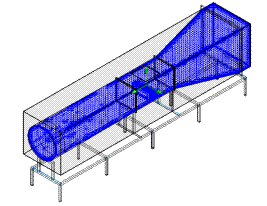Figure 4. 3D mesh.

3. Results and Discussion

The software "SolidWorks Flow Simulation" has been used to present the local characteristics. The numerical model considered is based on the resolution of the Navier-Stokes equations in conjunction with the standard k-ε turbulence model. These equations were solved by a finite volume discretization method [19-21].

3.1. Magnitude Velocity

Figure 5 presents the distribution of the magnitude velocity in the longitudinal plane defined by X=0 mm. According to these results, it has been noted that the velocity is weak in the inlet of the collector. It is indeed governed by the boundary conditions value of the inlet velocity which is equal to 0.9 m.s-1. In this region, the velocity field is found to be uniform and increases progressively downstream of the collector. At the test vein, an important increase has been noted due to the reduction of the tunnel section. While the upper side of the obstacle is characterized by the high velocity, a brutal drop is located behind the obstacle and this is due to the deceleration of the velocity while passing through the obstacle. In the test vein, the velocity keeps increasing until the out of the test section. Then, a decrease has been noted through the diffuser where the minimum velocity values are recorded in the lateral walls of the diffuser. In the considered gap located between the test vein surface and the obstacle, a wake characteristic of the maximum values has been observed. The study of the height effect shows that the velocity increases with the obstacle height. In fact, with a height obstacle h=H the velocity is equal to V=8 m.s-1. However, it is equal to V=18.4 m.s-1 with a h=2H.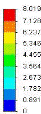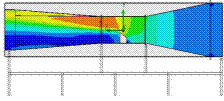(a) h= H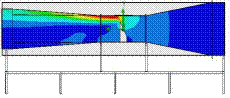(b) h=2 H

Figure 5. Distribution of the magnitude velocity.

3.2. Velocity Streamlines

Figure 6 presents the distribution of the velocity streamlines in the longitudinal plane defined by X=0 mm. According to these results, it has been noted that the velocity is weak in the inlet of the collector. In this region, the velocity is found to be uniform and increases progressively downstream of the collector. At the test vein, an important increase has been noted due to the reduction of the tunnel section that causes the throttling of the flow. While the upper side of the obstacle is characterized by the high velocity, a brutal drop is located behind the obstacle. This fact is due to the deceleration of the velocity field while passing through the obstacle. In the test vein, the velocity keeps increasing till the out of the test section. Then, a decrease of the maximum values has been noted through the diffuser where the minimum velocity values are recorded in the lateral walls of the diffuser. Indeed, a circulation zone has been observed behind the obstacle. In the considered gap, a wake characteristic of the maximum values appears between the test vein surface and the obstacle. In addition, we have noted that the velocity streamlines increases with the obstacle height. In fact, with a high obstacle h=H, the velocity is equal to V=8 m.s-1. However, it is equal to V=18.4 m.s-1with h=2H. Indeed, it has been noted that the recirculation zone is larger with the height obstacle h=2H.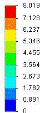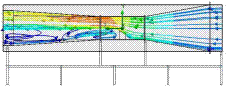(a) h= H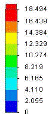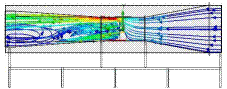(b) h=2 H

Figure 6. Distribution of the velocity streamlines.

3.3. Static Pressure

Figure 7 presents the distribution of the static pressure in the longitudinal plane defined by X=0 mm. According to these results, it has been noted that the static pressure is on its maximum in the inlet of the collector. Besides, it has been observed a depression above the obstacle. The pressure continues decreasing the way out of the test vein. A brutal drop of the pressure has been noted just behind the obstacle. The distribution of the static pressure shows that the depression zone characteristic of the minimum values is located in the second half of the wind tunnel through the diffuser. This is due to the dead zone in that area which is more important with h=2H. In these conditions, the static pressure is equal to p=101315.6 Pa with the height roof obstacle and it is equal to p=101200.4 Pa with h=2H.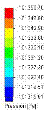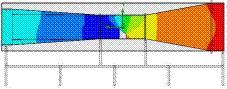(a) h= H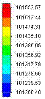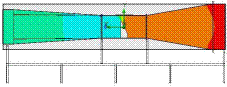(b) h=2 H

Figure 7. Distribution of the static pressure.

3.4. Dynamic Pressure

Figure 8 presents the distribution of the dynamic pressure in the longitudinal plane defined by X=0 mm. According to these results, the dynamic pressure is found to be weak in the collector inlet and increases gradually through the collector as long as the tunnel section gets smaller. When it gets to the test section, the dynamic pressure keeps increasing in the upstream of the obstacle. A compression zone is recorded in the region located behind the obstacle and is developed through the diffuser. However, a depression zone is located in the downside of the wind tunnel. This fact is more important in the region behind the obstacle with a height h=H than h= 2H.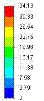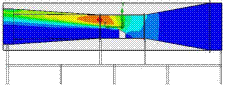(a) h= H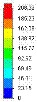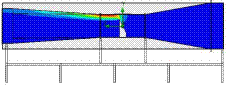(b) h=2 H

Figure 8. Distribution of the dynamic pressure.

3.5. Turbulent Kinetic Energy

Figure 9 presents the distribution of the turbulent kinetic energy in the longitudinal plane defined by X=0 mm. From these results, it has been noted that the turbulent kinetic energy is found to be very weak in the first half of the wind tunnel in the obstacle upstream. A wake characteristic of the maximum value of the turbulent kinetic energy appears in the obstacle upstream. This wake starts in the obstacle corner until the outlet of the diffuser. In addition, it has been noted that the maximum value of the turbulent kinetic energy increases with the obstacle height. In fact, with a height obstacle h=H, the turbulent kinetic energy is equal to k=2.4 m2.s-2. However, it is equal to k=20 m2.s-2with h= 2H.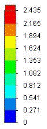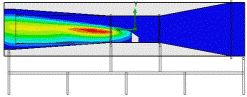(a) h= H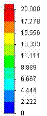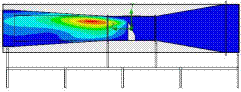(b) h=2 H

Figure 9. Distribution of the turbulent kinetic energy

3.6. Turbulent Dissipation Rate

Figure 10 presents the distribution of the turbulent dissipation rate in the longitudinal plane defined by X=0 m. From these results, it has been noted that the turbulent dissipation rate is found to be very weak in the first half of the wind tunnel and in the obstacle upstream. The wake characteristic of the maximum value of the turbulent dissipation rate appears upstream of the obstacle. The wake starts in the obstacle corner until the outlet of the diffuser. In addition, it is clear that the maximum value of the turbulent dissipation rate increases with the increase of the obstacle height. In fact, with an obstacle height h=H the turbulent dissipation rate is equal to ε=38.9 W/Kg. However, it is equal to ε=582.7 W/Kg with h=2H.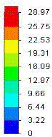(a) h= H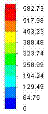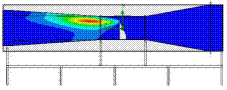(b) h=2 H

Figure 10. Distribution of the turbulent dissipation rate.

3.7. Turbulent Viscosity

Figure 11 presents the distribution of the turbulent viscosity in the longitudinal plane defined by X=0 mm. According to these results, it has been observed that the viscosity is at its minimum in the collector region and starts increasing after crossing the obstacle. Its maximum is located in the outlet of the diffuser. In addition, it has been noted that the turbulent height viscosity increases with the increase of the obstacle height. In fact, with an obstacle height h=H the turbulent viscosity is equal to μt=0.05 Pa.s. However, it is equal to μt=0.08 Pa.s with h= 2H.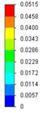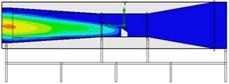(a) h= H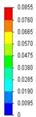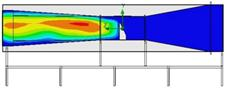(b) h=2 H

Figure 11. Distribution of turbulent viscosity.

3.8. Vorticity

Figure 12 presents the distribution of the vorticity in the longitudinal plane defined by X=0 mm. According to these results, the vorticity is at its minimum in the collector region and starts increasing after crossing the obstacle. The greatest vorticity values are reached after hitting the obstacle Corner. Indeed, it has been noted that the vorticity increases with the obstacle height. In fact, with an obstacle height h=H, the maximum value of vorticity is equal to 70 s-1. However, it is equal to 207.7 s-1 with h=2H.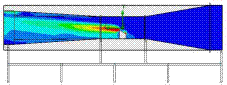(a) h= H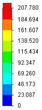(b) h=2 H

Figure 12. Distribution of vorticity.

4. Comparison with Experimental Results

The wind tunnel results are used to validate the CFD model. The experiment is carried out in the atmospheric boundary layer wind tunnel at the National school of Engineering of Sfax, Tunisia. The simulated data are interpolated at the same grid points as in the wind tunnel experiment (figure 13). As the configuration is replicated from the wind tunnel experiment, the CFD model tested using the mathematical model is validated against the data obtained from the wind tunnel experiments. The velocity profiles are chosen for points situated in the test section. The considered planes are defined by Z=0 mm, Z=150 mm and Z= -150 mm. For each transverse planes, values are taken along the directions defined by X=0 mm. The results for each plane are shown in figure 14. According to these profiles, it has been noted that the velocity value is very weak near the obstacle. Outside, the velocity has a maximum value. The comparison between the numerical and experimental results leads us to the conclusion that despite some unconformities, the values are comparable. The numerical model seems to be able to predict the aerodynamic characteristics of the air flow around the obstacle.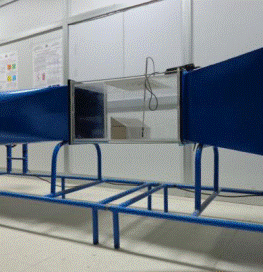Figure 13. Wind tunnel.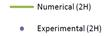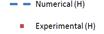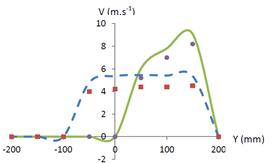(a) Z=0 mm and X=0 mm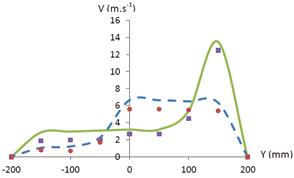(b) Z=150 mm and X=0 mm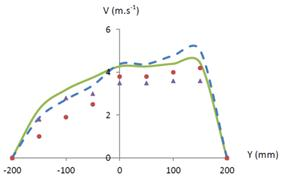(c) z=-150 mm and x=0 mm

Figure 14. Velocity profils.

5. Conclusion

In this work, we have developed a numerical simulation to study the height effect of an inclined roof obstacle on the aerodynamic characteristics. Numerical results, such as velocity fields, pressure and turbulent characteristics are presented in the wind tunnel. According to the numerical results, it has been noted that the roof obstacle shape has a direct effect on the local characteristics. The study of the height effect shows that the velocity increases with the obstacle height and the recirculation zones become larger. Also, the maximum values of the turbulent characteristics increase with the obstacle height. Use of this knowledge will assist the design of packaged installations of the wind rotors in the buildings. In the future, we propose to apply this study on the building design.

References

1. Nozawa, K., Tamura, I.N., 2002, Large eddy simulation of the flow around a low-rise building immersed in a rough-wall turbulent boundary layer , Journal of Wind Engineering and Industrial Aerodynamics, 90, 1151-1162.
2. Ikhwan, M., Ruck, B., 2006, Flow and pressure field characteristics around pyramidal buildings, Journal of Wind Engineering and Industrial Aerodynamics, 94 745-765.
3. Ahmad, K., Khare, M., Chaudhry, K.K., 2005, Wind tunnel simulation studies on dispersion at urban street canyons and intersections-a review, Journal of Wind Engineering and Industrial Aerodynamics, 90, 697-717.
4. De Melo, A. M. V., Santos, J. M., Mavrroidis, I. , Reis Junior, N. C., 2012, Modelling of odour dispersion around a pig farm building complex using AERMOD and CALPUFF. Comparison with wind tunnel results, Building and Environment, 56, 8-20.
5. Tominaga, Y., Stathopoulos, T., 2013, CFD simulation of near-field pollutant dispersion in the urban environment: A review of current modeling techniques, Atmospheric Environment, 79, 716-730.
6. Ould said, B., Retiel, N., Bouguerra, E.H., 2014, Numerical Simulation of Natural Convection in a Vertical Conical Cylinder Partially Annular Space, American Journal of Energy Research, 2(2), 24-29.
7. Princevac, M. Baik, J. Li, X., Pan, H., Park, S. , 2010, Lateral channeling within rectangular arrays of cubical obstacles, Journal of Wind Engineering and Industrial Aerodynamics, 98, 377-385.
8. Lateb, M., Masson, C., Stathopoulos, T., Bédard, C., 2013, Comparison of various type of k- ε models for pollutant emissions around a two-building configuration, Journal of Wind Engineering and Indusrial Aerodynamics, 115, 9-21.
9. Vizotto, I., Computational generation of free-form shells in architectural design and civil engineering, Automation in construction, 19, 1087-1105
10. Jiang, Y., Alexander, D., Jenkins, H., Arthur, R., Chen, Q., 2003, Natural ventilation in buildings: measurement in a wind tunnel and numerical simulation with large-eddy simulation, Journal of Wind Engineering and Industrial Aerodynamics, 91, 331-353.
11. Fabien, J.R., Martin, H., Jacob, W., Computer Simulation Of Driving Rain On Building Envelopes, 2nd European and African Conference on Wind Engineering, Genova, 22-26 June 1997.
12. Lim, H.C., Thomas, T.G., Castro, I.P., 2009, Flow around a cube in a turbulent boundary layer: LES and experiment, Journal of Wind Engineering and Industrial Aerodynamics, 97, 96-109.
13. Ntinas, G.K., Zhangb, G., Fragos, V.P., Bochtis, D.D., Nikita-Martzopoulou, C., 2014, Airflow patterns around obstacles with arched and pitched roofs: Wind tunnel measurements and direct simulation, European Journal of Mechanics B/Fluids, 43, 216-229.
14. Luo, W., Dong, Z., Qian, G., Lu, J., 2012, Wind tunnel simulation of the three-dimensional airflow patterns behind cuboid obstacles at different angles of wind incidence and their significance for theformation of sand shadows, Geomorphology, 139-140, 258-270.
15. Rafailidis, S., Schatzmann, M., 1995, Concentration Measurements with Different Roof Patterns in Street Canyon with Aspect Ratios B/H¼1/2 and B/H¼1, Universität Hamburg, Meterologisches Institute.
16. Driss, Z., Bouzgarrou, G., Chtourou, W., Kchaou, H., Abid, M.S., 2010, Computational studies of the pitched blade turbines design effect on the stirred tank flow characteristics, European Journal of Mechanics B/Fluids, 29, 236-245.
17. Ammar, M., Chtourou, W., Driss, Z., Abid, M.S., 2011, Numerical investigation of turbulent flow generated in baffled stirred vessels equipped with three different turbines in one and two-stage system, Energy, 36, 5081-5093.
18. Driss, Z., Abid, M.S., 2012, Use of the Navier-Stokes Equations to Study of the Flow Generated by Turbines Impellers. Navier-Stokes Equations: Properties, Description and Applications, 3, 51-138.
19. Driss, S, Driss, Z, Kallel Kammoun, I, 2014, Study of the Reynolds Number Effect on the Aerodynamic Structure around an Obstacle with Inclined Roof, Sustainable Energy, 2( 4), 126-133.
20. Driss, Z., Ammar, M., Chtourou, W., Abid, M.S., 2011, CFD Modelling of Stirred Tanks. Engineering Applications of Computational Fluid Dynamics, 5, 145-258.

 Contents 1. 2. 2.1. 2.2. 2.3. 3. 3.1. 3.2. 3.3. 3.4. 3.5. 3.6. 3.7. 3.8. 4. 5.
Article ToolsAbstractPDF(1972K)